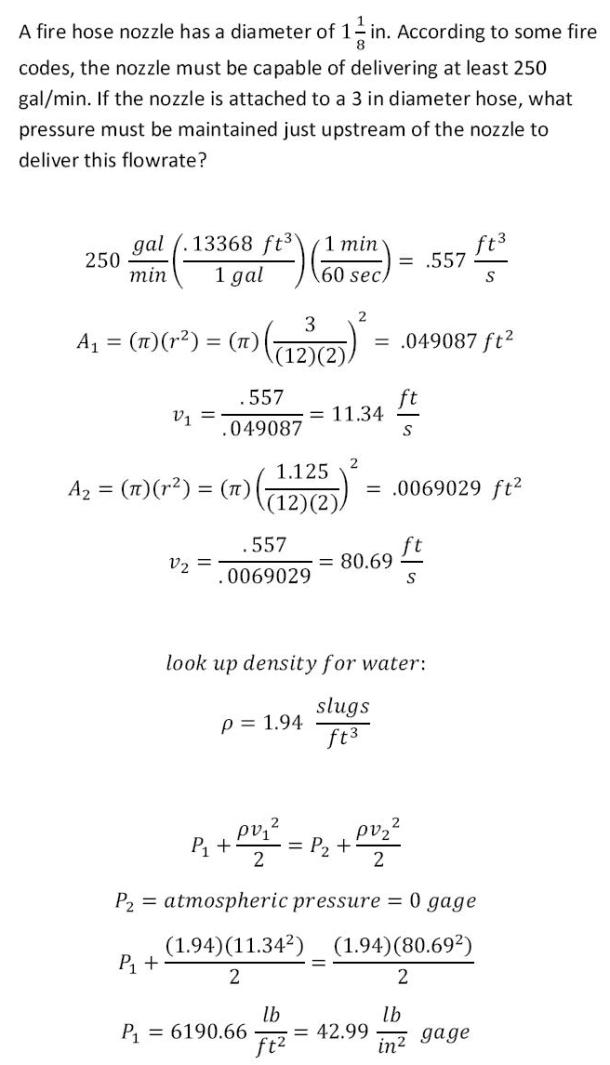fluid mechanics math problems engineering equations fluid mechanics basic mechanics school homework engineering math fluids formulas fluid mechanics problems fluid mechanics problem solutions to fluids problems full solution fluid mechanics engineering fluids problem solution fluid mechanics math problems engineering equations fluid mechanics basic mechanics school homework engineering math fluid mechanics formulas fluid problems fluid mechanics problem solutions to fluid mechanics problems full solution fluid mechanics engineering fluid mechanics problem solution
fluid mechanics math problems engineering equations fluid mechanics basic mechanics school homework engineering math fluids formulas fluid mechanics problems fluid mechanics problem solutions to fluids problems full solution fluid mechanics engineering fluids problem solution fluid mechanics math problems engineering equations fluid mechanics basic mechanics school homework engineering math fluid mechanics formulas fluid problems fluid mechanics problem solutions to fluid mechanics problems full solution fluid mechanics engineering fluid mechanics problem solution
Highalphabet Home Page fluid mechanics solutions fluids math solved Fluid Mechanics Page 1
A fire hose nozzle has a diameter of 1 1/8 in. According to some fire codes, the nozzle must be capable of delivering at least 250 gal/min. If the nozzle is attached to a 3 in diameter hose, what pressure must sbe maintained just upstream of the nozzle to deliver this flowrate?A fire hose nozzle has a diameter of 1 1/8 in. According to some fire codes, the nozzle must be capable of delivering at least 250 gal/min. If the nozzle is attached to a 3 in diameter hose, what pressure must sbe maintained just upstream of the nozzle to deliver this flowrate?